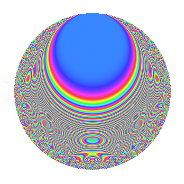# Properties

 Label 403.2.vLevel $403$ Weight $2$ Character orbit 403.v Rep. character $\chi_{403}(36,\cdot)$ Character field $\Q(\zeta_{6})$ Dimension $70$ Newform subspaces $1$ Sturm bound $74$ Trace bound $0$

# Related objects

## Defining parameters

 Level: $$N$$ $$=$$ $$403 = 13 \cdot 31$$ Weight: $$k$$ $$=$$ $$2$$ Character orbit: $$[\chi]$$ $$=$$ 403.v (of order $$6$$ and degree $$2$$) Character conductor: $$\operatorname{cond}(\chi)$$ $$=$$ $$403$$ Character field: $$\Q(\zeta_{6})$$ Newform subspaces: $$1$$ Sturm bound: $$74$$ Trace bound: $$0$$

## Dimensions

The following table gives the dimensions of various subspaces of $$M_{2}(403, [\chi])$$.

Total New Old
Modular forms 78 78 0
Cusp forms 70 70 0
Eisenstein series 8 8 0

## Trace form

 $$70q - 6q^{2} + 4q^{3} + 30q^{4} + 6q^{6} - 12q^{7} + 58q^{9} + O(q^{10})$$ $$70q - 6q^{2} + 4q^{3} + 30q^{4} + 6q^{6} - 12q^{7} + 58q^{9} - q^{10} - 6q^{11} + 13q^{12} - 14q^{13} - 14q^{14} - 15q^{15} - 28q^{16} + 6q^{17} + 12q^{19} + 9q^{21} - 8q^{22} + 10q^{23} + 19q^{25} + 34q^{27} - 18q^{29} - 31q^{30} + 2q^{31} + 36q^{32} - 12q^{33} - 9q^{34} - 12q^{35} + 8q^{36} - 21q^{38} - 30q^{39} + 5q^{40} + 18q^{41} - 49q^{42} + 19q^{43} - 42q^{44} - 63q^{45} - 6q^{46} - 27q^{48} + 9q^{49} - 7q^{51} - 43q^{52} - 22q^{53} + 18q^{54} + 30q^{55} + 25q^{56} - 15q^{57} - 12q^{58} + 33q^{59} - 13q^{61} - 17q^{62} - 6q^{63} - 38q^{64} + 9q^{65} - 52q^{66} + 30q^{67} + 88q^{68} - 16q^{69} + 9q^{73} - 19q^{74} + 25q^{75} + 34q^{77} + 14q^{78} + 6q^{79} + 6q^{80} + 22q^{81} - 78q^{82} + 54q^{83} - 33q^{85} + 24q^{86} - 14q^{87} + 16q^{88} - 6q^{89} - 11q^{90} - 70q^{91} - 6q^{92} + 7q^{93} - 43q^{94} + 25q^{95} - 36q^{96} - 75q^{97} - 93q^{99} + O(q^{100})$$

## Decomposition of $$S_{2}^{\mathrm{new}}(403, [\chi])$$ into newform subspaces

Label Dim. $$A$$ Field CM Traces $q$-expansion
$$a_2$$ $$a_3$$ $$a_5$$ $$a_7$$
403.2.v.a $$70$$ $$3.218$$ None $$-6$$ $$4$$ $$0$$ $$-12$$